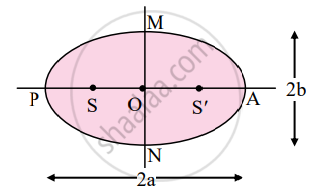# Answer the following question in detail. State Kepler’s three laws of planetary motion. - Physics

Short Note

Answer the following question in detail.

State Kepler’s three laws of planetary motion.

#### Solution

1. Kepler’s law of orbits:

Statement:
All planets move in elliptical orbits around the Sun with the Sun at one of the foci of the ellipse.2. Kepler’s law of equal areas:

Statement:
The line that joins a planet and the Sun sweeps equal areas in equal intervals of time.3. Kepler’s law of periods:

Statement:
The square of the time period of revolution of a planet around the Sun is proportional to the cube of the semimajor axis of the ellipse traced by the planet.

Concept: Kepler’S Laws
Is there an error in this question or solution?

#### APPEARS IN

Balbharati Physics 11th Standard Maharashtra State Board
Chapter 5 Gravitation
Exercises | Q 3. (xiii) | Page 98